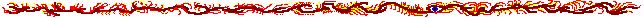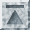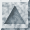# fdgrid.h [plain] [printer]

```#ifndef __FDGRID_H__
#define __FDGRID_H__

#define NODEBUG

#include <mpi++.h>
#include <magic/object.h>
#include <magic/Matrix.h>

/** Processor topology handling tool.
*
*  Handy for determining neighbouring processors.
**/
class ProcessorGrid {
public:
ProcessorGrid   (int q) : mQ (q) {}

int     row         (int id) const {return id/mQ;}
int     column      (int id) const {return id%mQ;}

int     leftPid     (int id) const {return ((id%mQ) > 0)?    (id-1)  : MPI_PROC_NULL;}
int     rightPid    (int id) const {return ((id%mQ) < mQ-1)? (id+1)  : MPI_PROC_NULL;}
int     upPid       (int id) const {return ((id/mQ) > 0)?    (id-mQ) : MPI_PROC_NULL;}
int     downPid     (int id) const {return ((id/mQ) < mQ-1)? (id+mQ) : MPI_PROC_NULL;}

private:
int     mQ;
};

/** Two-dimensional finite difference grid segment, globally acting.
*
*  This is a globalized solution to finite difference calculation,
*  where the data is represented as a real-valued matrix, not as an
*  object grid as it would be in a localized solution. The reason for
*  using a globalized solution comes from the MPI_Type_vector
*  datatype, which can be used to easily transmit values of matrices,
*  but not of objects.
**/
class FDGridSegment : public Object {
public:
/** Constructor.
*
*  @param N Size of the entire grid is N*N.
*
*  @param mpi The global MPI instance for communication.
**/
FDGridSegment   (int N, MPIInstance& mpi);

/** Executes the finite difference calculation until termination
*  criteria is met.
**/
void            execute         (int maxcycles, double epsilon);

protected:
/** Initialization of a datapoint. Must be implemented. Must
*  return the initial value of point (r,c), where r and c are
*  absolute coordinates in the full matrix.
*
*  Notice that the point coordinated may be outside the
*  computation matrix area (0,0)-(N-1,N-1). These data points are
*  in the "outside" edge of the data. They will not be calculated
*  or otherwise changed later, but they *must* be set here, or
*  they will be invalid.
**/
virtual double  initPoint       (int r, int c) const=0;

/** Computes a new value for a data point. Must be
*  implemented. Must return the update value for point (r,c),
*  where r and c are absolute coordinates in the full matrix.
*
*  Notice that the implementor does not need to know *anything*
*  about the process borders or the outern edge of the matrix.
**/
virtual double  compute         (int r, int c) const=0;

inline double   value           (int r, int c) const {
ASSERTWITH (r>=mRow0-1 && r<=mRow1+1 && c>=mCol0-1 && c<=mCol1+1,
format ("Process %d may only access values in area (%d,%d)-(%d,%d),"
" point (%d,%d) was out of range.\n",
mrMPI.world().getRank(), mRow0,mCol0,mRow1,mCol1,r,c));
return mMatrix.get(r-mRow0+1, c-mCol0+1);
}

/** Overload this to add functionality at the start of every cycle. */
virtual void    startOfCycle    () {}

/** Overload this to add functionality at the end of every cycle. */
virtual void    endOfCycle      () {}

/** Returns the current iteration cycle. */
int             cycle           () const {return mCycle;}

/** Returns the size of the N*N matrix. */
int             N               () const {return mN;}

MPIInstance&    mpi             () {return mrMPI;}

private:
/** Number of elements in the matrix in both direction; N*N. */
int             mN;

/** Initializes the grid segment. */
void            init            ();

/** The data matrix plus ghost points for communication with
*  neighbour processes.
**/
Matrix          mMatrix;

/** Current iteration cycle. */
int             mCycle;
MPIInstance&    mrMPI;
ProcessorGrid   mProcessorGrid;
int             mRow0;
int             mRow1;
int             mCol0;
int             mCol1;
MPIVector       mColumnVectorType;
};

#endif
```Generated with (Python version) of tab4.cgi by Marko Grönroos (magi@iki.fi), 1999